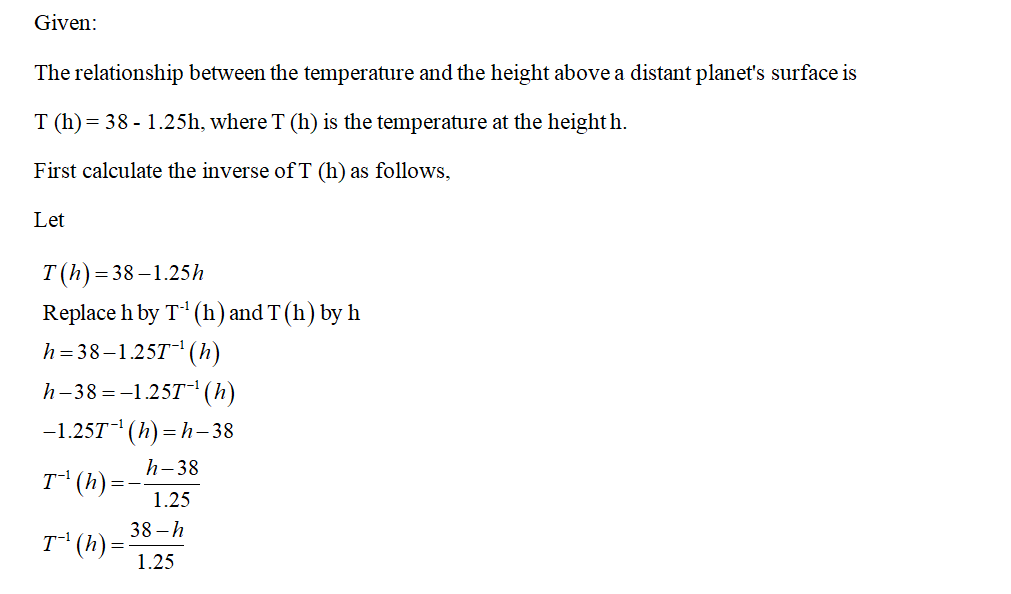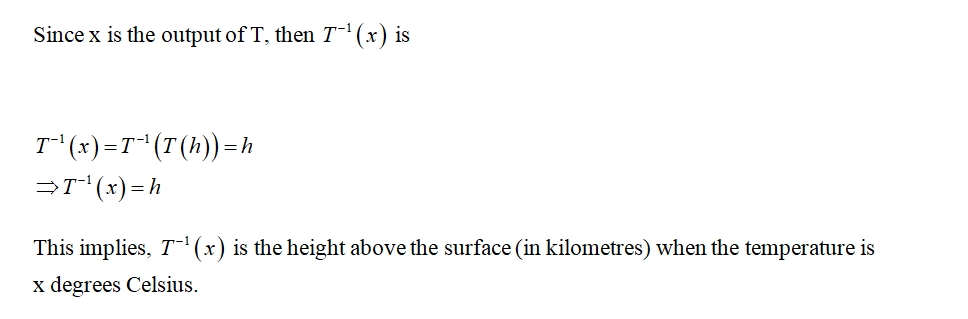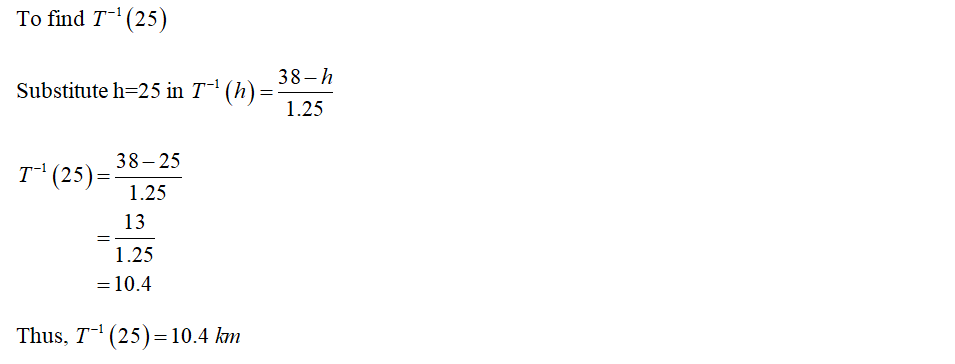# Scientists have found a relationship between the temperature and the height above a distant planet's surface. T(h), given below, is the temperature in Celsius at a height of h kilometers above the planet's surface. The relationship is as follows.T(h) = 38 - 1.25hComplete the following statements.Let T−1 be the inverse function of T.Take x to be an output of the function T.(a) Which statement best describes T^-1(x)?  The reciprocal of the temperature (in degrees Celsius) at a height of x kilometers.  The height above the surface (in kilometers) when the temperature is x degrees Celsius.  The temperature (in degrees Celsius) at a height of x kilometers.  The ratio of the temperature (in degrees Celsius) to the number of kilometers, x. (b) T^-1(x)= (c) T^-1(25)=

Question
26 views
Scientists have found a relationship between the temperature and the height above a distant planet's surface.
T(h), given below, is the temperature in Celsius at a height of
h kilometers above the planet's surface. The relationship is as follows.
T(h) = 38 - 1.25h
Complete the following statements.
 Let T−1 be the inverse function of T.Take x to be an output of the function T. (a) Which statement best describes T^-1(x)? The reciprocal of the temperature (in degrees Celsius) at a height of x kilometers. The height above the surface (in kilometers) when the temperature is x degrees Celsius. The temperature (in degrees Celsius) at a height of x kilometers. The ratio of the temperature (in degrees Celsius) to the number of kilometers, x. (b) T^-1(x)= (c) T^-1(25)=

check_circle

Step 1Step 2Step 3...

### Want to see the full answer?

See Solution

#### Want to see this answer and more?

Solutions are written by subject experts who are available 24/7. Questions are typically answered within 1 hour.*

See Solution
*Response times may vary by subject and question.
Tagged in